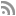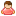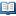## Active Neurons 2

1,2051,00012# Transistor achievement in Active Neurons 2

Transistor

To invent the First Transistor-8.2
2 guides## How to unlock the Transistor achievement

•Dwaggienite2,563,567
15 Sep 2020 15 Sep 2020 19 Sep 2020
13 5 9
The Solutions:
Remember the numbers for each shape.

11-1: L U D R U R (input numbers) R U L D L L U

11-2: D L U R U R D D L U D L L L L U D R U (input numbers) U U D L R U D L D R U R R L L U U D R

11-3: U L L U R D R U U L D L U R D L D R U R R R L L L D L U R D D L D R U R R R L L U (input numbers) U (room 2) L U D R U R U L D U D U U U L D R U U U L L D D L U U R U R D L L R U R L D R R U R L D

11-4: L D D R D L U R D L D R R U L R U L U R D U R D R (input numbers) R

11-5: U R D L U U U L D R R D R U U L U D R U U L D L U U R U L U (input numbers) U

11-6: U L D R D R D L D L U L D R R U D L U R U L U L D R D R R U L U R L D R U R (input numbers) R

11-7: D R U L D D R D L U U L U R R R L L L R U R D L D D D L D R R R L U U (input numbers) U U L U

11-8: L D R R U R U L U L L D L D L D D R U U L D R U L R (input numbers) U L L

11-9: D R U U D L D R R U U L U R U R D L U U R L U U R U D R D L L U U R U L D L U L U (input numbers) U

11-10: R D L D R U L U R (remember numbers in following sequence) U L R U D R U D R L (end sequence to attain numeric code) D L U R D L U L U (wait for yellow block to destroy red) D R U L (enter code from "ULRUDRUDRL sequence)

Guide not helping? View 1 more guide for this achievement.11-10 Working Solution

RDLDRULUR- (remember numbers in following sequence)
ULRUDRUDRL- (end sequence to attain numeric code)
DLURDLULU- (wait for yellow block to destroy red)
DRUL- (enter code from "ULRUDRUDRL sequence)
Posted by CuddIe Bunny on 17 Sep at 17:23Thank you Cuddle Bunny. You can't see it, but I have actually put the UPs in there. For some reason, the solution isn't showing them. It might be a TA solution error in regards to too many button pictures in one solution. I've changed the solution to show your solution instead, since button-presses don't seem to show up.
Posted by Dwaggienite on 18 Sep at 10:18For 11-9: Had better luck with 11-9: D R U U D L D R R U U L U R U R D L U U D R D L L U U R U L D L U L U (input numbers) U

The 'R L U U R U' bit gets you stuck in the upper left corner.
Posted by VidGamiac on 03 Oct at 06:09
• Have you got any tips or tricks to unlock this achievement?
Add a guide to share them with the community.

•Cheevo Guides262,018
09 Sep 2020 08 Sep 2020 08 Sep 2020
3 2 0
This game is longer than the first one. This one has 120 Levels. You get an achievement every 10 levels. I think the average person will take 2-3 hours with a guide. You can also pressto view the solution. However, you will have to memorize it. Or you can watch the video guide📺🏆